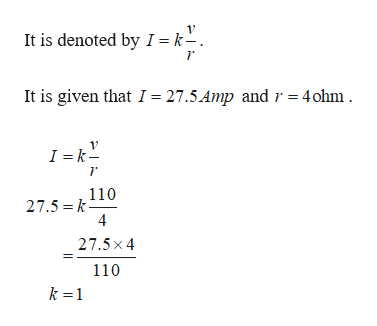# The current (I) in a wire varies directly as the voltage (v) and inversely as the resistance (r). If the current is 27.5 amps when the voltage is 110 volts and the resistance is 4 ohms, find the current when the voltage is 180 volts and the resistance is 16 ohms. (Round your answer to two decimal places.)amps

Question
18 views

The current (I) in a wire varies directly as the voltage (v) and inversely as the resistance (r). If the current is 27.5 amps when the voltage is 110 volts and the resistance is 4 ohms, find the current when the voltage is 180 volts and the resistance is 16 ohms. (Round your answer to two decimal places.)
amps

check_circle

Step 1

It is given that the current (I) is varies directly a...help_outlineImage TranscriptioncloseIt is denoted by I = k-. It is given that I = 27.5 Amp and r 4ohm I = k- 110 27.5 k 4 27.5x 4 110 k 1 fullscreen

### Want to see the full answer?

See Solution

#### Want to see this answer and more?

Solutions are written by subject experts who are available 24/7. Questions are typically answered within 1 hour.*

See Solution
*Response times may vary by subject and question.
Tagged in

### Other